# Polynomials

13 Aug 2013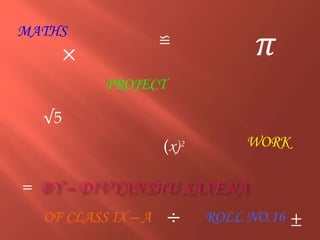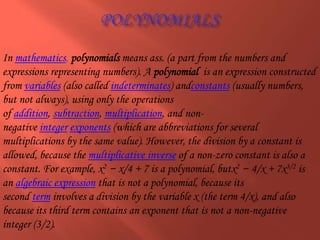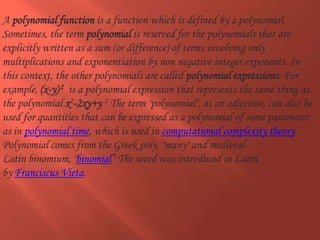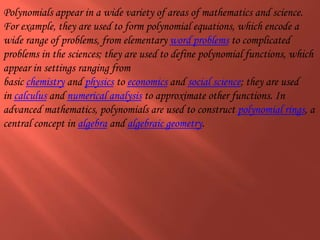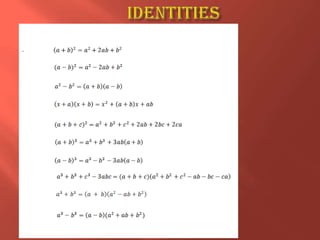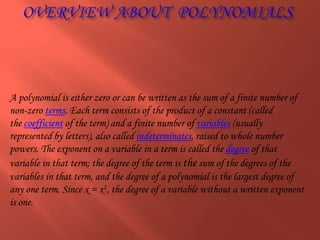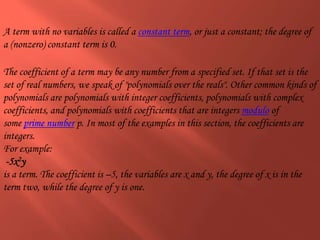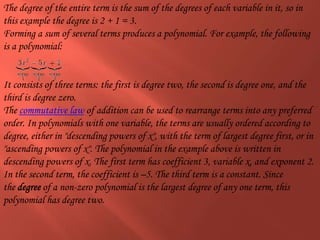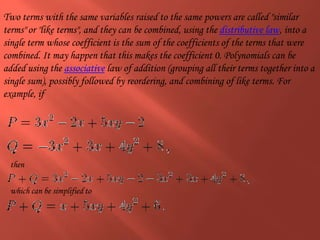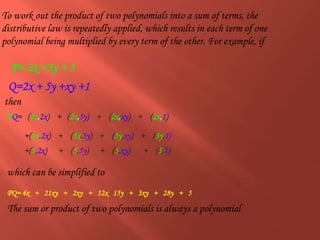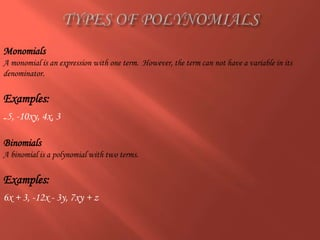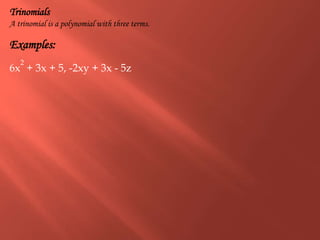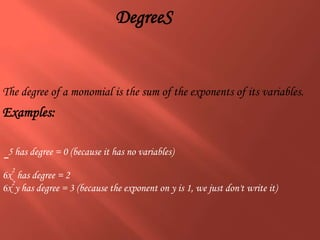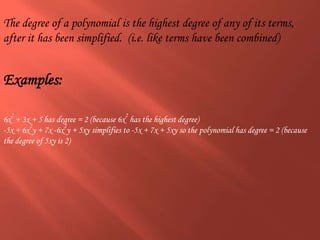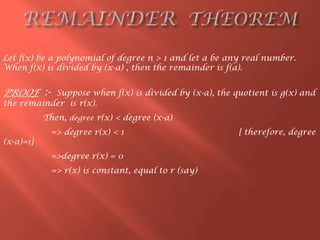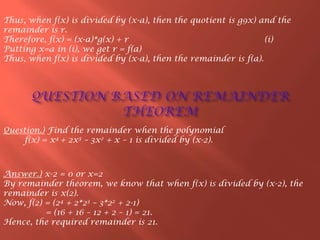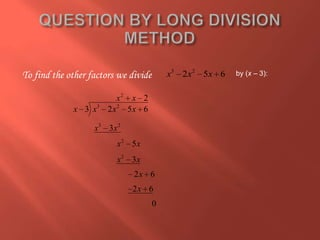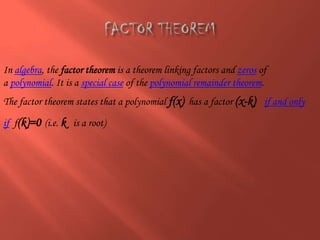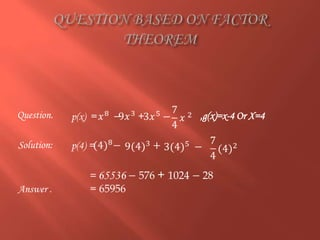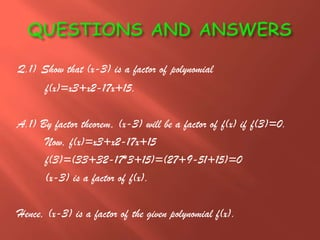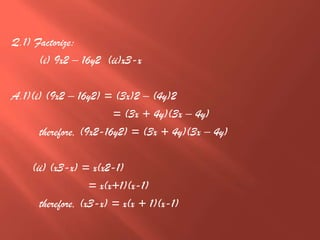1 sur 23

### Polynomials

• 1. MATHS PROJECT WORK OF CLASS IX – A ROLL NO.16 (x)2
• 2. INDEX  1).POLYNOMIALS.  2).IDENTITIES.  3).OVERVIEW ABOUT POLYNOMIALS.  4).TYPES OF POLYNOMIALS.  5).ABOUT DEGREES. POLYNOMIALS
• 3. In mathematics, polynomials means ass. (a part from the numbers and expressions representing numbers). A polynomial is an expression constructed from variables (also called indeterminates) andconstants (usually numbers, but not always), using only the operations of addition, subtraction, multiplication, and non- negative integer exponents (which are abbreviations for several multiplications by the same value). However, the division by a constant is allowed, because the multiplicative inverse of a non-zero constant is also a constant. For example, x2 − x/4 + 7 is a polynomial, butx2 − 4/x + 7x3/2 is an algebraic expression that is not a polynomial, because its second term involves a division by the variable x (the term 4/x), and also because its third term contains an exponent that is not a non-negative integer (3/2).
• 4. A polynomial function is a function which is defined by a polynomial. Sometimes, the term polynomial is reserved for the polynomials that are explicitly written as a sum (or difference) of terms involving only multiplications and exponentiation by non negative integer exponents. In this context, the other polynomials are called polynomial expressions. For example, (x-y)2 is a polynomial expression that represents the same thing as the polynomial x2-2xy+y 2 The term "polynomial", as an adjective, can also be used for quantities that can be expressed as a polynomial of some parameter, as in polynomial time, which is used in computational complexity theory. Polynomial comes from the Greek poly, "many" and medieval Latin binomium, "binomial” The word was introduced in Latin by Franciscus Vieta.
• 5. Polynomials appear in a wide variety of areas of mathematics and science. For example, they are used to form polynomial equations, which encode a wide range of problems, from elementary word problems to complicated problems in the sciences; they are used to define polynomial functions, which appear in settings ranging from basic chemistry and physics to economics and social science; they are used in calculus and numerical analysis to approximate other functions. In advanced mathematics, polynomials are used to construct polynomial rings, a central concept in algebra and algebraic geometry.
• 6. .
• 7. A polynomial is either zero or can be written as the sum of a finite number of non-zero terms. Each term consists of the product of a constant (called the coefficient of the term) and a finite number of variables (usually represented by letters), also called indeterminates, raised to whole number powers. The exponent on a variable in a term is called the degree of that variable in that term; the degree of the term is the sum of the degrees of the variables in that term, and the degree of a polynomial is the largest degree of any one term. Since x = x1, the degree of a variable without a written exponent is one.
• 8. The coefficient of a term may be any number from a specified set. If that set is the set of real numbers, we speak of "polynomials over the reals". Other common kinds of polynomials are polynomials with integer coefficients, polynomials with complex coefficients, and polynomials with coefficients that are integers modulo of some prime number p. In most of the examples in this section, the coefficients are integers. For example: -5x2y is a term. The coefficient is –5, the variables are x and y, the degree of x is in the term two, while the degree of y is one. A term with no variables is called a constant term, or just a constant; the degree of a (nonzero) constant term is 0.
• 9. The degree of the entire term is the sum of the degrees of each variable in it, so in this example the degree is 2 + 1 = 3. Forming a sum of several terms produces a polynomial. For example, the following is a polynomial: It consists of three terms: the first is degree two, the second is degree one, and the third is degree zero. The commutative law of addition can be used to rearrange terms into any preferred order. In polynomials with one variable, the terms are usually ordered according to degree, either in "descending powers of x", with the term of largest degree first, or in "ascending powers of x". The polynomial in the example above is written in descending powers of x. The first term has coefficient 3, variable x, and exponent 2. In the second term, the coefficient is –5. The third term is a constant. Since the degree of a non-zero polynomial is the largest degree of any one term, this polynomial has degree two.
• 10. Two terms with the same variables raised to the same powers are called "similar terms" or "like terms", and they can be combined, using the distributive law, into a single term whose coefficient is the sum of the coefficients of the terms that were combined. It may happen that this makes the coefficient 0. Polynomials can be added using the associative law of addition (grouping all their terms together into a single sum), possibly followed by reordering, and combining of like terms. For example, if then which can be simplified to
• 11. To work out the product of two polynomials into a sum of terms, the distributive law is repeatedly applied, which results in each term of one polynomial being multiplied by every term of the other. For example, if then which can be simplified to The sum or product of two polynomials is always a polynomial PQ= 4x + 21xy + 2xy + 12x 15y + 3xy + 28y + 5 P= 2x +3y + 5 PQ= (2x.2x) + (2x.5y) + (2x.xy) + (2x.1) +(3y.2x) + (3x.5y) + (3y.xy) + (3y.1) +(5.2x) + (5.5y) + (5.xy) + (5.1) Q=2x + 5y +xy +1
• 12. Monomials A monomial is an expression with one term. However, the term can not have a variable in its denominator. Examples: -5, -10xy, 4x, 3 Binomials A binomial is a polynomial with two terms. Examples: 6x + 3, -12x - 3y, 7xy + z
• 13. Trinomials A trinomial is a polynomial with three terms. Examples: 6x 2 + 3x + 5, -2xy + 3x - 5z
• 14. The degree of a monomial is the sum of the exponents of its variables. Examples: -5 has degree = 0 (because it has no variables) 6x 2 has degree = 2 6x 2 y has degree = 3 (because the exponent on y is 1, we just don't write it) DegreeS
• 15. The degree of a polynomial is the highest degree of any of its terms, after it has been simplified. (i.e. like terms have been combined) Examples: 6x 2 + 3x + 5 has degree = 2 (because 6x 2 has the highest degree) -5x + 6x 2 y + 7x -6x 2 y + 5xy simplifies to -5x + 7x + 5xy so the polynomial has degree = 2 (because the degree of 5xy is 2)
• 16. PROOF :- Suppose when f(x) is divided by (x-a), the quotient is g(x) and the remainder is r(x). Then, degree r(x) < degree (x-a) => degree r(x) < 1 [ therefore, degree (x-a)=1] =>degree r(x) = 0 => r(x) is constant, equal to r (say) Let f(x) be a polynomial of degree n > 1 and let a be any real number. When f(x) is divided by (x-a) , then the remainder is f(a).
• 17. Thus, when f(x) is divided by (x-a), then the quotient is g9x) and the remainder is r. Therefore, f(x) = (x-a)*g(x) + r (i) Putting x=a in (i), we get r = f(a) Thus, when f(x) is divided by (x-a), then the remainder is f(a). Answer.) x-2 = 0 or x=2 By remainder theorem, we know that when f(x) is divided by (x-2), the remainder is x(2). Now, f(2) = (24 + 2*23 – 3*22 + 2-1) = (16 + 16 – 12 + 2 – 1) = 21. Hence, the required remainder is 21. Question.) Find the remainder when the polynomial f(x) = x4 + 2x3 – 3x2 + x – 1 is divided by (x-2).
• 18. 3 2 2 5 6x x x 2 3 2 3 2 2 2 2 3 2 5 6 3 5 3 2 6 2 6 0 x x x x x x x x x x x x x x To find the other factors we divide by (x – 3):
• 19. In algebra, the factor theorem is a theorem linking factors and zeros of a polynomial. It is a special case of the polynomial remainder theorem. The factor theorem states that a polynomial f(x) has a factor (x-k) if and only if f(k)=0 (i.e. k is a root)
• 21. Q.1) Show that (x-3) is a factor of polynomial f(x)=x3+x2-17x+15. A.1) By factor theorem, (x-3) will be a factor of f(x) if f(3)=0. Now, f(x)=x3+x2-17x+15 f(3)=(33+32-17*3+15)=(27+9-51+15)=0 (x-3) is a factor of f(x). Hence, (x-3) is a factor of the given polynomial f(x).
• 22. Q.1) Factorize: (i) 9x2 – 16y2 (ii)x3-x A.1)(i) (9x2 – 16y2) = (3x)2 – (4y)2 = (3x + 4y)(3x – 4y) therefore, (9x2-16y2) = (3x + 4y)(3x – 4y) (ii) (x3-x) = x(x2-1) = x(x+1)(x-1) therefore, (x3-x) = x(x + 1)(x-1)